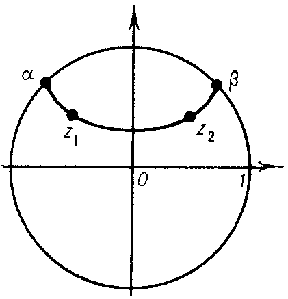# Pick theorem

Schwarz' lemma in invariant form

The following generalization of the Schwarz lemma. Let $w = f( z)$ be a bounded regular analytic function in the unit disc $\Omega = \{ {z \in \mathbf C } : {| z | < 1 } \}$, $| f( z) | \leq 1$ for $| z | < 1$. Then for any points $z _ {1}$ and $z _ {2}$ in $\Omega$ the non-Euclidean distance $d( w _ {1} , w _ {2} )$ of their images $w _ {1} = f( z _ {1} )$ and $w _ {2} = f( z _ {2} )$ does not exceed the non-Euclidean distance $d( z _ {1} , z _ {2} )$, i.e.

$$\tag{1 } d( w _ {1} , w _ {2} ) \leq d( z _ {1} , z _ {2} ) ,\ \ | z _ {1} | , | z _ {2} | < 1.$$

One also has the inequality

$$\tag{2 } \frac{| dw | }{1- | w | ^ {2} } \leq \frac{| dz | }{1- | z | ^ {2} } ,\ \ dw = f ^ { \prime } ( z) dz,\ | z | < 1,$$

between the elements of non-Euclidean length (the differential form of Pick's theorem or the Schwarz lemma). Equality applies in (1) and (2) only if $w = f( z)$ is a Möbius function that maps $\Omega$ onto itself (cf. Fractional-linear mapping).

The non-Euclidean, or hyperbolic, distance $d( z _ {1} , z _ {2} )$ is the distance in Lobachevskii geometry between $z _ {1}$ and $z _ {2}$ when $\Omega$ is the Lobachevskii plane and arcs of circles serve as Lobachevskii straight lines, these being orthogonal to the unit circle (Poincaré's model), and

$$d( z _ {1} , z _ {2} ) = \frac{1}{2} \mathop{\rm ln} ( \alpha , \beta , z _ {1} , z _ {2} ) =$$

$$= \ \frac{1}{2} \mathop{\rm ln} \frac{| 1- \overline{z}\; _ {2} z _ {2} | +| z _ {1} - z _ {2} | }{| 1- \overline{z}\; _ {1} z _ {2} | - | z _ {1} - z _ {2} | } ,$$

where $( \alpha , \beta , z _ {1} , z _ {2} )$ is the cross ratio between the points $z _ {1}$ and $z _ {2}$ and the points of intersection $\alpha$ and $\beta$ of the Lobachevskii straight line passing through $z _ {1}$ and $z _ {2}$ with the unit circle (see Fig.).Figure: p072700a

The non-Euclidean length of the image $f( L)$ of any rectifiable curve $L \subset \Omega$ under the mapping $w = f( z)$ does not exceed the non-Euclidean length of $L$.

The theorem was established by G. Pick ; a far-reaching generalization of it is provided by the principle of the hyperbolic metric (cf. Hyperbolic metric, principle of the). In geometric function theory these theorems provide bounds for various functionals related to mapping functions , .

How to Cite This Entry:
Pick theorem. Encyclopedia of Mathematics. URL: http://encyclopediaofmath.org/index.php?title=Pick_theorem&oldid=48179
This article was adapted from an original article by E.D. Solomentsev (originator), which appeared in Encyclopedia of Mathematics - ISBN 1402006098. See original article